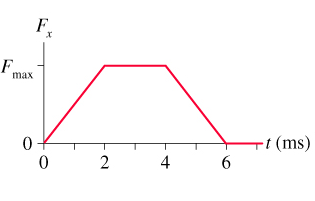# Problem: A 60g tennis ball with an initial speed of 32 m/s hits a wall and rebounds with the same speed. The figure shows the force of the wall on the ball during the collision. What is the value of Fmax, the maximum value of the contact force during the collision? (2 sig figs)

###### FREE Expert Solution

Impulse:

$\overline{){\mathbf{I}}{\mathbf{=}}{\mathbf{F}}{\mathbf{t}}}$

Change in momentum:

$\overline{){\mathbf{∆}}{\mathbf{P}}{\mathbf{=}}{{\mathbf{mv}}}_{{\mathbf{f}}}{\mathbf{-}}{{\mathbf{mv}}}_{{\mathbf{0}}}}$

Impulse is equal to force under the curve:

I = (1/2)bh + l•w + (1/2)bh = (1/2)(2 - 0)Fmax + (4 - 2)Fmax + (1/2)(6 - 2)Fmax = 4Fmax N.ms

I = 4Fmax N.ms (10-3 s/ 1ms) = 0.004Fmax N•s

Change in momentum:

96% (342 ratings)###### Problem Details

A 60g tennis ball with an initial speed of 32 m/s hits a wall and rebounds with the same speed. The figure shows the force of the wall on the ball during the collision. What is the value of Fmax, the maximum value of the contact force during the collision? (2 sig figs)